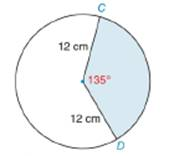Chapter 8.5, Problem 18E### Elementary Geometry for College St...

6th Edition
Daniel C. Alexander + 1 other
ISBN: 9781285195698

#### Solutions

Chapter
Section### Elementary Geometry for College St...

6th Edition
Daniel C. Alexander + 1 other
ISBN: 9781285195698
Textbook Problem
1 views

# Find the exact perimeter and area of the sector shown.To determine

To find:

The exact perimeter and area of the sector.

Explanation

Definition:

Arc Measure:

In a circle, the degree measure of an arc is equal to the measure of central angle that intercepts the arc.

Formula:

Perimeter of sector:

If r is the radius of circle,

Psector=r+r+lintercepted arc=2r+lintercepted arc

Thus, Psector=2r+lintercepted arc

Area of sector:

If r is the radius of the circle, the area A of a sector whose arc has degree measure m is given by

A=m360×πr2

Length of an arc:

In a circle whose circumference is C, the length l of an arc whose degree measure is m is given by:

l=m360×C

We know that, circumference of a circle is given by 2πr where r is the radius of circle.

Hence, length l of an arc =m360×2πr

Calculation:

Perimeter of sector:

From the diagram it is clear that radius of the circle is 12 cm.

The measure of central angle is given as 1350.

As per the definition of measure of an arc, the degree measure of an arc is equal to the central angle intercepted by it.

Thus, the degree measure of arc CD^ =1350. Hence, m=1350

Length l of an arc is given by the formula m360×2πr

Let’s substitute the value of m and r to find the length of arc CD^

### Still sussing out bartleby?

Check out a sample textbook solution.

See a sample solution

#### The Solution to Your Study Problems

Bartleby provides explanations to thousands of textbook problems written by our experts, many with advanced degrees!

Get Started

#### Find more solutions based on key concepts